Understanding Interactions of Acids and Bases

Understanding Interactions of Acids and Bases

Key Terms

• Strong (acid or base)
• Weak (acid or base)
• Conjugate acid
• Conjugate base
• Lewis base
• Lewis acid
• Lewis acid/Lewis base complex

Objectives

• Use different acid/base models to identify acids and bases
• Evaluate the basic characteristics and interactions of acids and bases

Acid and Base Models

We consider three models of acids and bases: the Arrhenius, Brønsted-Lowry, and Lewis models. These models differ most fundamentally in how they classify acids and bases, with each having a slightly different focus.

The Arrhenius model defines an acid as a substance that, when dissolved in water, ionizes to yield protons (H+), and it defines a base as a substance that, when dissolved in water, yields hydroxide ions (OH-). Thus, an acid HA (where A is some molecule) ionizes as follows in water: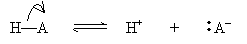The results of the ionization are a proton and an anion, A-. For a base XOH, the ionization follows the reaction below.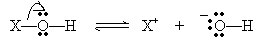This reaction yields a cation (X+) and a hydroxide ion (OH-). A strong acid or base ionizes completely in water; a weak acid or base does not ionize completely.

In the Brønsted-Lowry model, an acid is a proton donor and a base is a proton acceptor. Thus, an acid HA and a base B react as follows: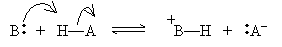The products BH+ and A- are called the conjugate acid and conjugate base, respectively. Note that if an acid (base) is strong, then its conjugate base (acid) must be weak, because the more an acid (base) tends to dissociate, the less its conjugate base (acid) tends to dissociate. The reverse is also true: a weak acid (base) has a strong conjugate base (acid).

But what causes an acid to be strong or weak? Several factors come into play. Consider a simple acid: a hydrogen halide (a hydrogen atom bonded to a halogen-fluorine, chlorine, and so on). If X is the halogen, then the acid HX tends to be strong if the bond between the halogen and the hydrogen is weak-that is, a proton is easily dissociated from the acid. As the atomic number of the halogen increases, the size of the atom also increases, causing a longer bond length with hydrogen. This increasing length decreases the bond strength, meaning that larger halogens form stronger acids when combined with hydrogen. Within a particular column of the periodic table, bond strength is the primary factor determining the strength of an acid.

A less influential consideration is the electronegativity of the elements involved. For hydrogen combined with elements in the third row of the periodic table (phosphorus, sulfur, and chlorine), for example, the increasing electronegativity of the atoms with increasing atomic number yields less of a hold on the protons owing to a stronger attraction of the electron pair in the bond. Thus, the protons (hydrogen ions) are more easily dissociated, meaning a stronger acid. H2S is therefore a weaker acid than HCl, for example. Within a particular row of the periodic table, electronegativity is the primary factor determining the strength of an acid.

Another factor influencing acid strength, but one we will not discuss at length, is electron delocalization. If the acid's conjugate base has resonance structures (electrons are delocalized among more than one bond), that conjugate base is more stable, meaning it is better able to exist in ionized form when it dissociates a proton.

The strength of an acid (or base) determines the favorableness of the ionization reaction involving that acid. We can express a reaction as favoring one set of products or another by emphasizing the arrow representing one direction over that of the other direction. For instance, given a strong acid HA in water, we can write the following: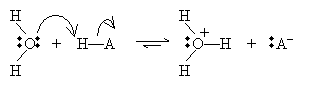In this case, the conjugate base (A-) is a weak base, so it does not readily accept a proton. Therefore, the reaction favors the products on the right, as indicated by the exaggerated rightward arrow.

The last acid/base model we will consider is the Lewis view. In this case, the focus is not on protons, but on electron pairs. An acid, according to the Lewis model, accepts an electron pair, whereas a base donates an electron pair. This view broadens the class of molecules that count as acids and bases to include many that do not contain or do not dissociate protons. A Lewis acid/base reaction may involve ions, as in the following, where H- is the Lewis base (an electron-pair donor) and OH+ is the Lewis acid (an electron-pair acceptor):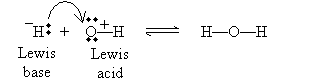or it may involve neutral reactants: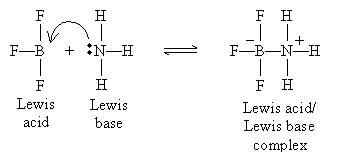Note that the product in this case (on the right side of the equation) is called a Lewis acid/Lewis base complex.

Practice Problem: Determine which of the following is a Lewis acid and which is a Lewis base.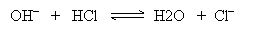Solution: One way to help identify the Lewis acid and Lewis base is to draw the movement of electrons in the reaction.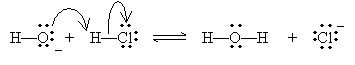The Lewis acid is the electron-pair acceptor: in this case, HCl. The Lewis base (the electron-pair donor) is the hydroxide ion (OH-).

Practice Problem: List the following Brønsted-Lowry acids in order of increasing strength.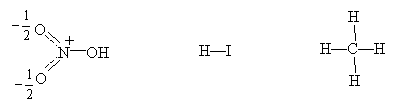Solution: A Brønsted-Lowry acid is a proton donor; thus, to determine the relative strength of each acid, we must consider how easily each molecule dissociates a proton. We can first note that carbon (in methane-CH4) and oxygen (in nitric acid-HNO3) are both bound to protons and both are in the second row of the periodic table. Thus, we know that because carbon is less electronegative than oxygen, nitric acid is stronger than methane. But what about hydrogen iodide (HI)? Remember that bond strength is a greater factor than electronegativity in determining acid strength; in this case, iodine is a much larger atom than oxygen, meaning that the bond length for the proton in HI is much longer than that in HNO3. Therefore, the proton is more easily dissociated from HI, making it the strongest of these three acids.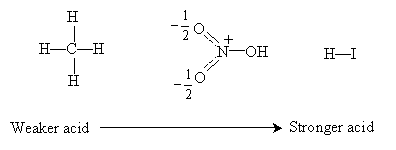Explore